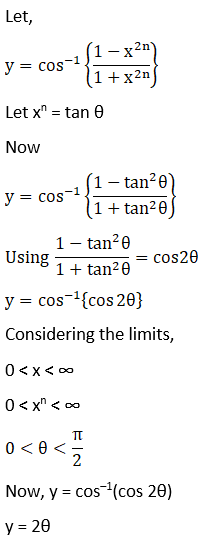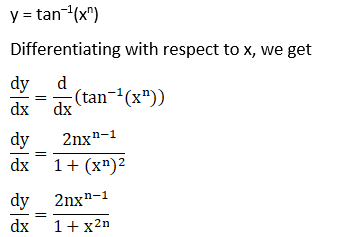# RD Sharma Solutions For Class 12 Maths Exercise 11.3 Chapter 11 Differentiation

RD Sharma Solutions for Class 12 Maths Exercise 11.3 Chapter 11 Differentiation is provided here for students to study and excel in their board exams. Differentiation by using trigonometrical substitutions is the main topic covered in this exercise. The solutions for RD Sharma book, solved by BYJU’S experts, explain the steps most easily without missing out on essential aspects of solving a question.

Students can easily view and download the RD Sharma Solutions for Class 12 Exercise 11.3 of Chapter 11 Differentiation from the below-provided links. These RD Sharma Solutions primarily improve their speed in solving problems, which builds time management skills, which is key from the exam perspective.

## RD Sharma Solutions for Class 12 Chapter 11 – Differentiation Exercise 11.3:

### Access other exercises of RD Sharma Solutions for Class 12 Chapter 11 – Differentiation

Exercise 11.1 Solutions

Exercise 11.2 Solutions

Exercise 11.4 Solutions

Exercise 11.5 Solutions

Exercise 11.6 Solutions

Exercise 11.7 Solutions

Exercise 11.8 Solutions

### Access answers to Maths RD Sharma Solutions for Class 12 Chapter 11 – Differentiation Exercise 11.3

Exercise 11.3 Page No: 11.62

Differentiate the following functions with respect to x: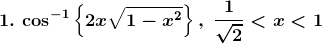Solution: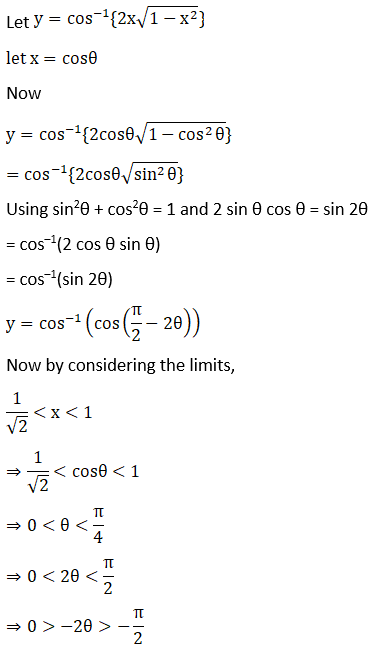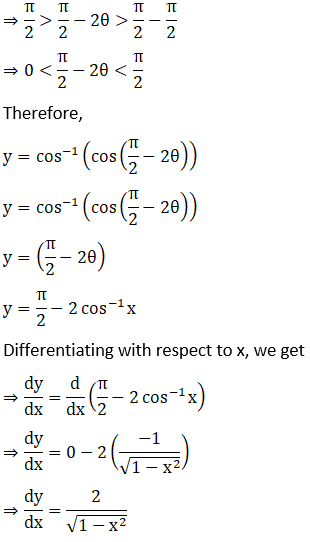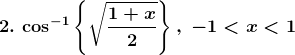Solution: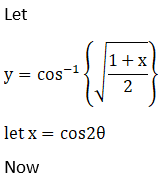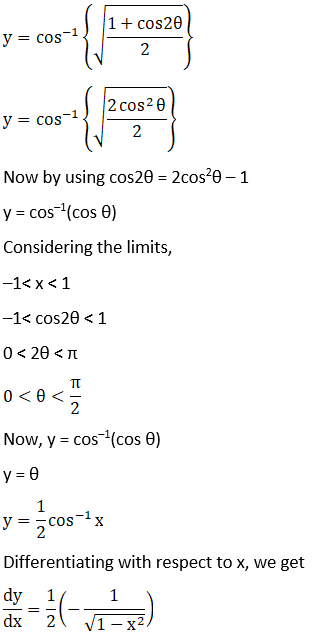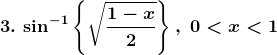Solution:

Let,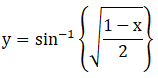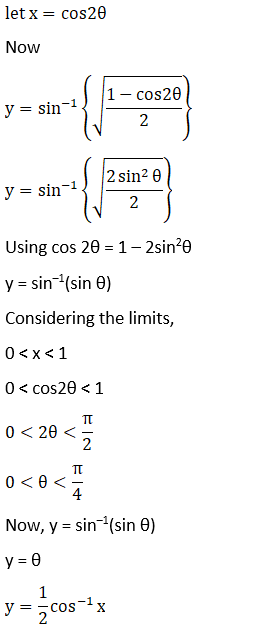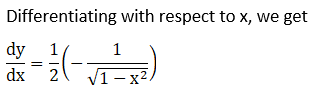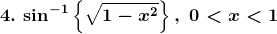Solution:

Let,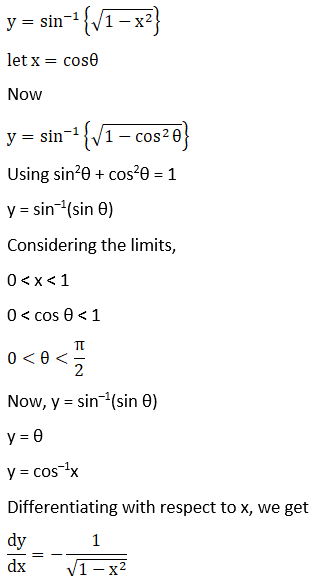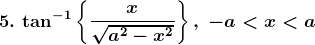Solution: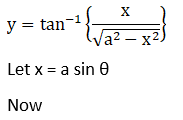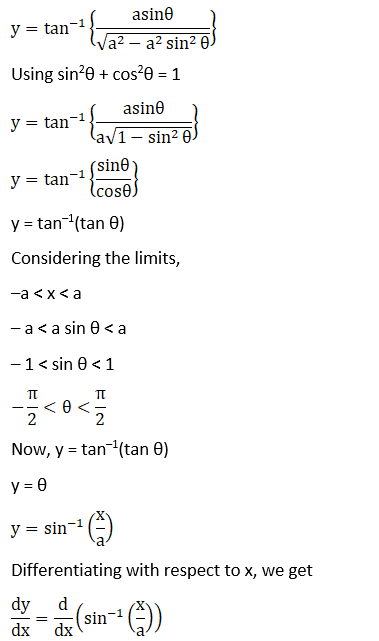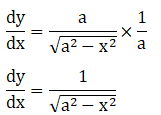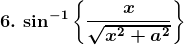Solution: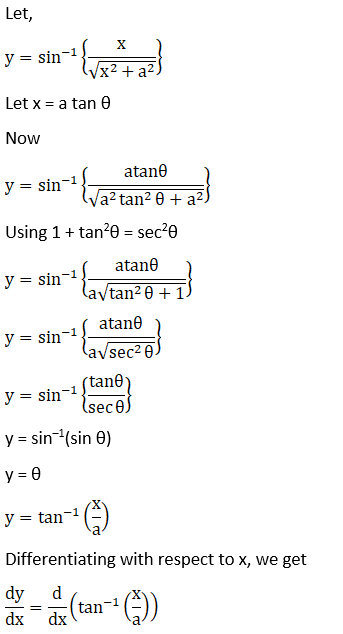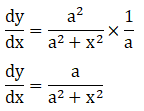7. Sin-1 (2x2 – 1), 0 < x < 1

Solution: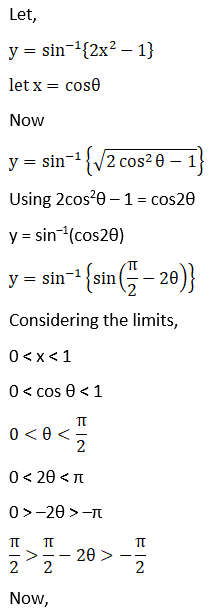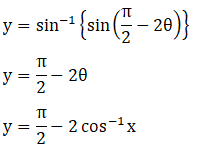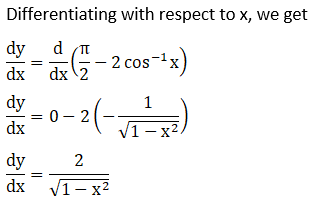8. Sin-1 (1 – 2x2), 0 < x < 1

Solution: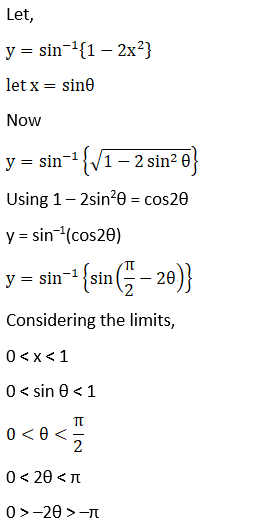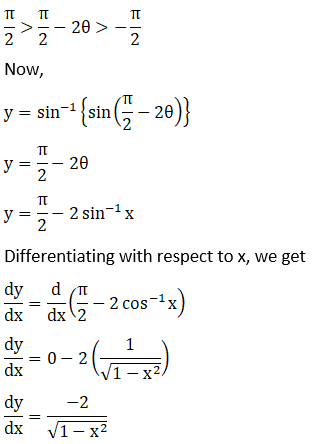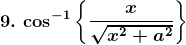Solution: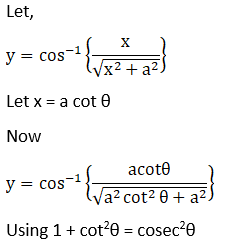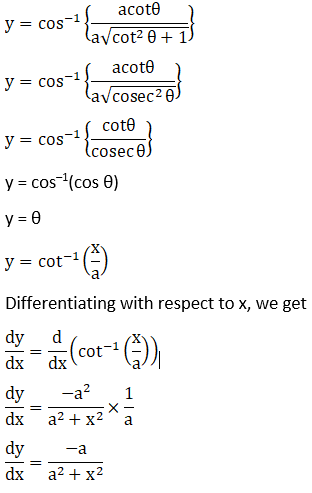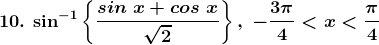Solution: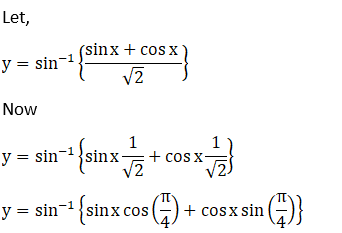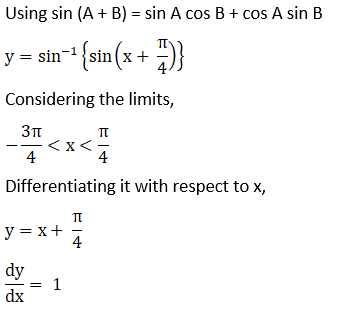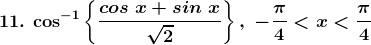Solution: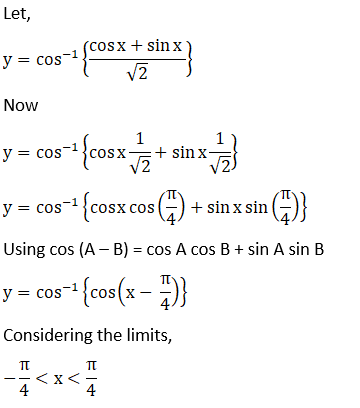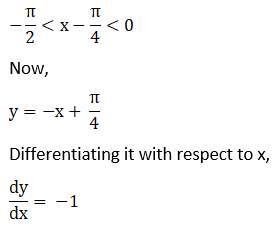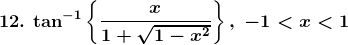Solution: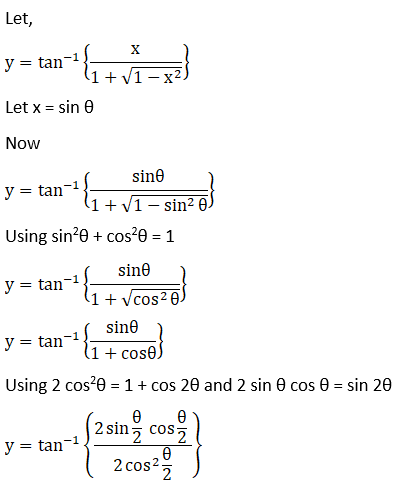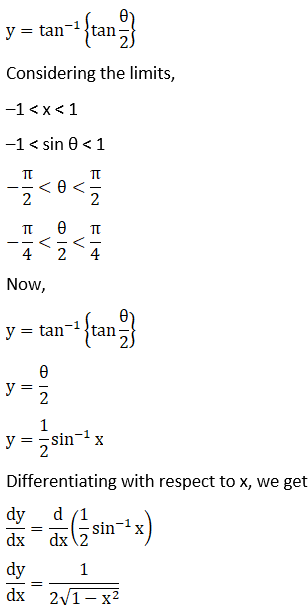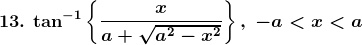Solution: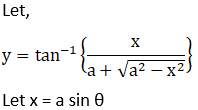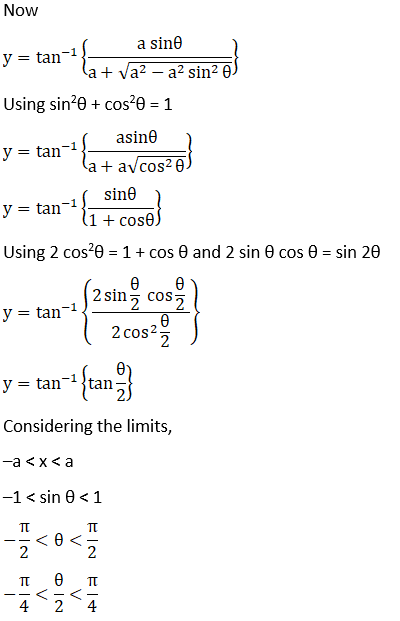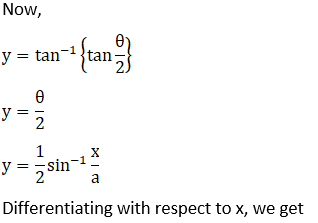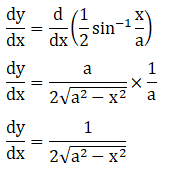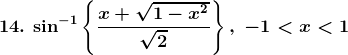Solution: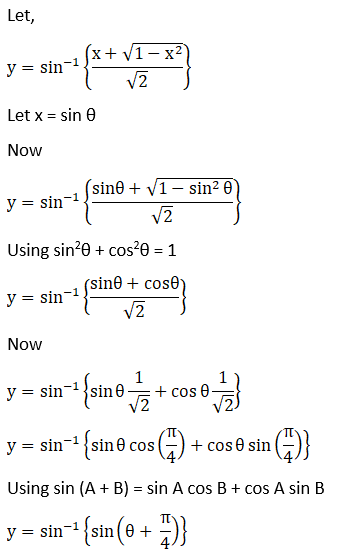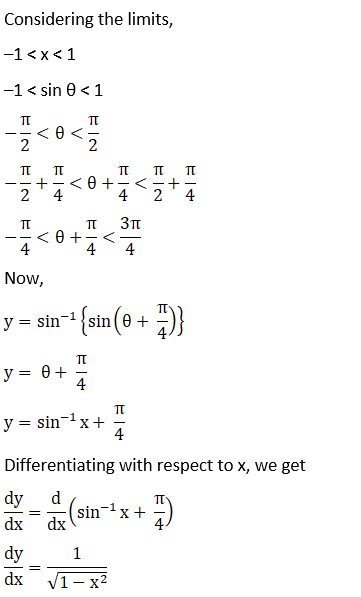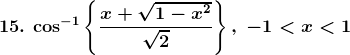Solution: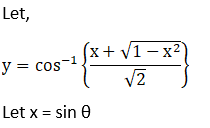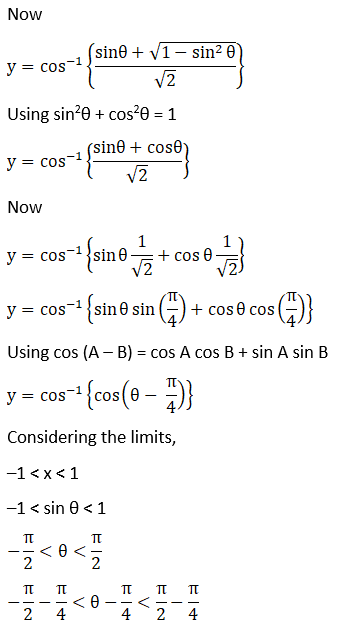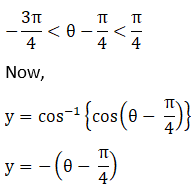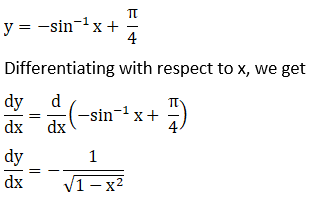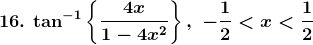Solution: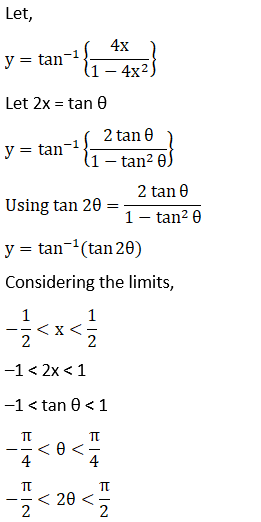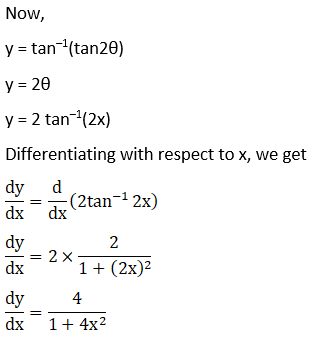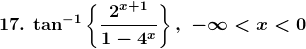Solution: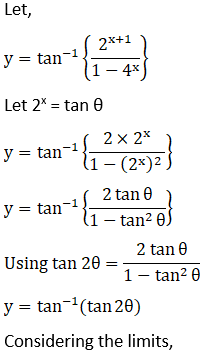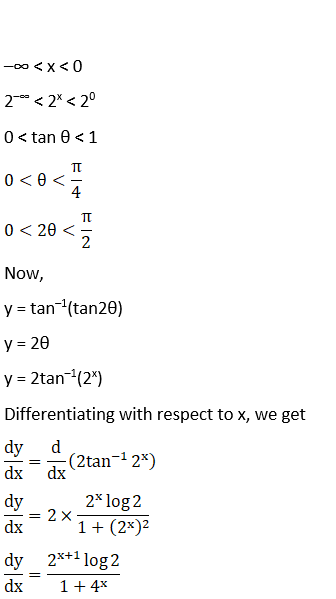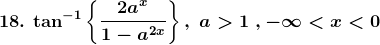Solution: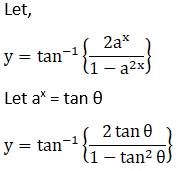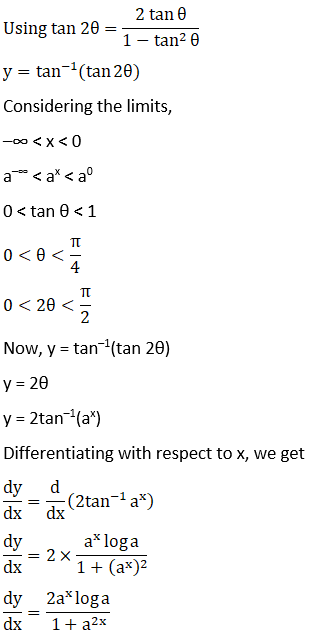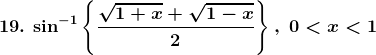Solution:

Let,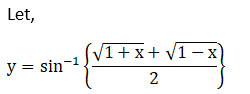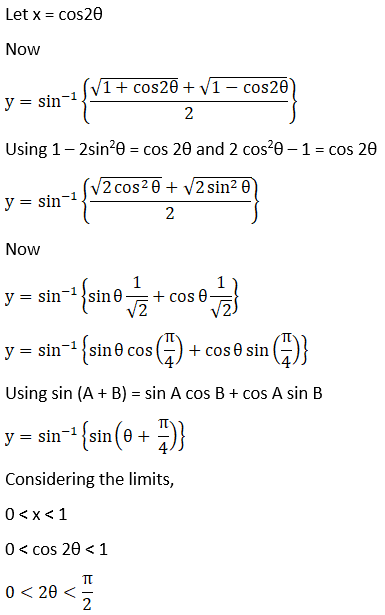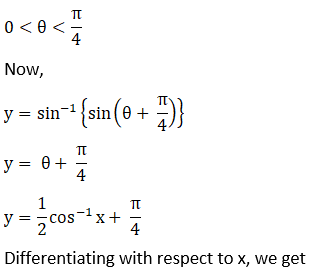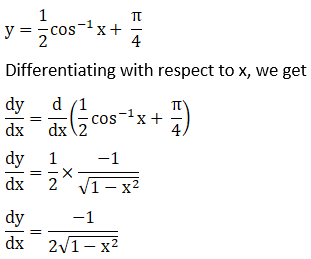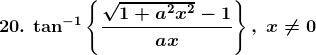Solution: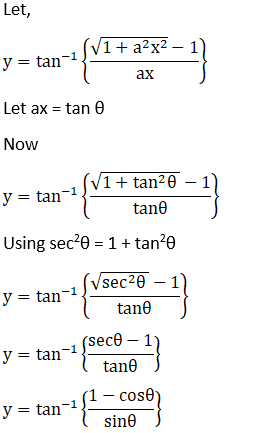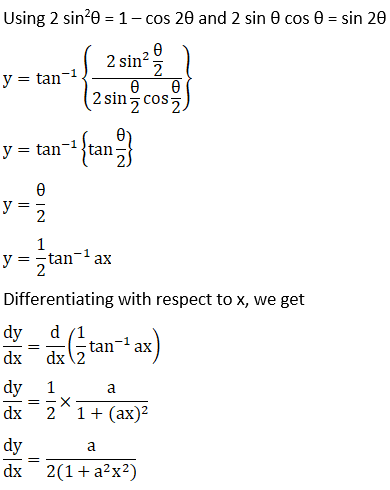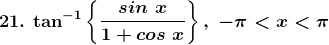Solution: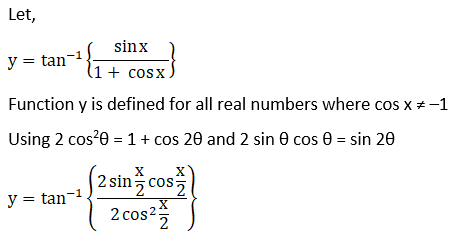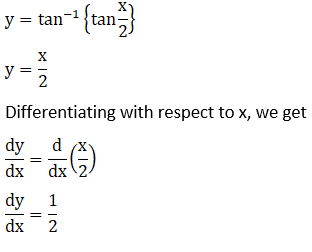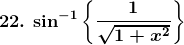Solution: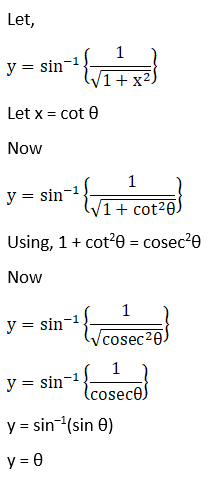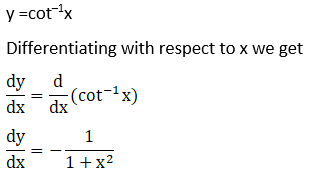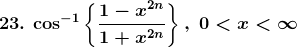Solution: